Question

Find the average rate of change of the function over the given interval.
Upper R (theta )equals StartRoot 4 theta plus 1 EndRoot​;​[2​,12​]

1.The average rate of change of the function over the given interval is of 0.4.

Step-by-step explanation:

The average rate of change of a function f(x) over an interval [a,b] is given by: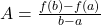In this question:

The function is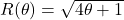, in the interval [2,12]. So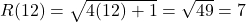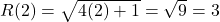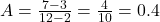The average rate of change of the function over the given interval is of 0.4.